# Mean State

Period Mean (original grids) [W/m2]
Model Period Mean (intersection) [W/m2]
Model Period Mean (complement) [W/m2]
Benchmark Period Mean (intersection) [W/m2]
Benchmark Period Mean (complement) [W/m2]
Bias [W/m2]
RMSE [W/m2]
Phase Shift [months]
Bias Score 
RMSE Score 
Seasonal Cycle Score 
Spatial Distribution Score 
Interannual Variability Score 
Overall Score 
Benchmark [-] 457.
CLM5PHSOFF [-] 464. 464. 0.00 456. 465. 7.94 16.8 1.52 0.43 0.38 0.79 0.97 0.63 0.60
CLM5PHSON [-] 463. 463. 0.00 456. 465. 7.83 16.4 1.44 0.46 0.38 0.80 0.98 0.61 0.60
Period Mean (original grids) [W/m2]
Model Period Mean (intersection) [W/m2]
Model Period Mean (complement) [W/m2]
Benchmark Period Mean (intersection) [W/m2]
Benchmark Period Mean (complement) [W/m2]
Bias [W/m2]
RMSE [W/m2]
Phase Shift [months]
Bias Score 
RMSE Score 
Seasonal Cycle Score 
Spatial Distribution Score 
Interannual Variability Score 
Overall Score 
Benchmark [-] 466.
CLM5PHSOFF [-] 484. 484. 0.00 467. 458. 17.4 23.4 0.546 0.46 0.51 0.95 0.91 0.65 0.67
CLM5PHSON [-] 484. 484. 0.00 467. 458. 17.2 23.5 0.637 0.46 0.50 0.94 0.95 0.62 0.66
Period Mean (original grids) [W/m2]
Model Period Mean (intersection) [W/m2]
Model Period Mean (complement) [W/m2]
Benchmark Period Mean (intersection) [W/m2]
Benchmark Period Mean (complement) [W/m2]
Bias [W/m2]
RMSE [W/m2]
Phase Shift [months]
Bias Score 
RMSE Score 
Seasonal Cycle Score 
Spatial Distribution Score 
Interannual Variability Score 
Overall Score 
Benchmark [-] 443.
CLM5PHSOFF [-] 454. 454. 0.00 443. 446. 11.6 18.9 1.04 0.52 0.50 0.86 1.0 0.64 0.67
CLM5PHSON [-] 452. 452. 0.00 443. 446. 9.13 18.8 1.38 0.57 0.46 0.81 1.0 0.60 0.65
Period Mean (original grids) [W/m2]
Model Period Mean (intersection) [W/m2]
Model Period Mean (complement) [W/m2]
Benchmark Period Mean (intersection) [W/m2]
Benchmark Period Mean (complement) [W/m2]
Bias [W/m2]
RMSE [W/m2]
Phase Shift [months]
Bias Score 
RMSE Score 
Seasonal Cycle Score 
Spatial Distribution Score 
Interannual Variability Score 
Overall Score 
Benchmark [-] 279.
CLM5PHSOFF [-] 281. 280. 0.00 278. 296. 2.48 15.5 0.126 0.90 0.81 0.99 1.0 0.75 0.88
CLM5PHSON [-] 277. 278. 0.00 278. 296. 2.37 15.4 0.127 0.90 0.81 0.99 1.0 0.74 0.88
Period Mean (original grids) [W/m2]
Model Period Mean (intersection) [W/m2]
Model Period Mean (complement) [W/m2]
Benchmark Period Mean (intersection) [W/m2]
Benchmark Period Mean (complement) [W/m2]
Bias [W/m2]
RMSE [W/m2]
Phase Shift [months]
Bias Score 
RMSE Score 
Seasonal Cycle Score 
Spatial Distribution Score 
Interannual Variability Score 
Overall Score 
Benchmark [-] 457.
CLM5PHSOFF [-] 459. 459. 0.00 454. 467. 4.79 14.7 1.53 0.29 0.38 0.77 0.66 0.58 0.51
CLM5PHSON [-] 459. 459. 0.00 454. 467. 5.54 14.6 1.55 0.29 0.38 0.77 0.65 0.58 0.51
Period Mean (original grids) [W/m2]
Model Period Mean (intersection) [W/m2]
Model Period Mean (complement) [W/m2]
Benchmark Period Mean (intersection) [W/m2]
Benchmark Period Mean (complement) [W/m2]
Bias [W/m2]
RMSE [W/m2]
Phase Shift [months]
Bias Score 
RMSE Score 
Seasonal Cycle Score 
Spatial Distribution Score 
Interannual Variability Score 
Overall Score 
Benchmark [-] 312.
CLM5PHSOFF [-] 318. 318. 0.00 311. 334. 6.89 12.9 0.0457 0.89 0.88 1.0 1.0 0.86 0.92
CLM5PHSON [-] 317. 317. 0.00 311. 334. 5.51 11.6 0.0521 0.91 0.88 1.0 1.0 0.86 0.92
Period Mean (original grids) [W/m2]
Model Period Mean (intersection) [W/m2]
Model Period Mean (complement) [W/m2]
Benchmark Period Mean (intersection) [W/m2]
Benchmark Period Mean (complement) [W/m2]
Bias [W/m2]
RMSE [W/m2]
Phase Shift [months]
Bias Score 
RMSE Score 
Seasonal Cycle Score 
Spatial Distribution Score 
Interannual Variability Score 
Overall Score 
Benchmark [-] 364.
CLM5PHSOFF [-] 369. 368. 0.00 363. 399. 5.34 14.6 0.138 0.85 0.82 0.99 1.0 0.76 0.87
CLM5PHSON [-] 366. 365. 0.00 363. 399. 4.07 14.2 0.164 0.86 0.82 0.99 1.0 0.76 0.87
Period Mean (original grids) [W/m2]
Model Period Mean (intersection) [W/m2]
Model Period Mean (complement) [W/m2]
Benchmark Period Mean (intersection) [W/m2]
Benchmark Period Mean (complement) [W/m2]
Bias [W/m2]
RMSE [W/m2]
Phase Shift [months]
Bias Score 
RMSE Score 
Seasonal Cycle Score 
Spatial Distribution Score 
Interannual Variability Score 
Overall Score 
Benchmark [-] 448.
CLM5PHSOFF [-] 457. 458. 0.00 446. 467. 11.6 19.4 0.677 0.57 0.57 0.93 0.98 0.64 0.71
CLM5PHSON [-] 453. 454. 0.00 446. 467. 9.50 18.9 0.825 0.60 0.56 0.91 0.98 0.63 0.71
Period Mean (original grids) [W/m2]
Model Period Mean (intersection) [W/m2]
Model Period Mean (complement) [W/m2]
Benchmark Period Mean (intersection) [W/m2]
Benchmark Period Mean (complement) [W/m2]
Bias [W/m2]
RMSE [W/m2]
Phase Shift [months]
Bias Score 
RMSE Score 
Seasonal Cycle Score 
Spatial Distribution Score 
Interannual Variability Score 
Overall Score 
Benchmark [-] 281.
CLM5PHSOFF [-] 285. 285. 0.00 281. 288. 4.57 13.4 0.0859 0.91 0.83 0.99 1.0 0.76 0.89
CLM5PHSON [-] 284. 284. 0.00 281. 288. 4.17 13.2 0.0840 0.91 0.83 0.99 1.0 0.77 0.89
Period Mean (original grids) [W/m2]
Model Period Mean (intersection) [W/m2]
Model Period Mean (complement) [W/m2]
Benchmark Period Mean (intersection) [W/m2]
Benchmark Period Mean (complement) [W/m2]
Bias [W/m2]
RMSE [W/m2]
Phase Shift [months]
Bias Score 
RMSE Score 
Seasonal Cycle Score 
Spatial Distribution Score 
Interannual Variability Score 
Overall Score 
Benchmark [-] 441.
CLM5PHSOFF [-] 447. 447. 0.00 443. 426. 4.58 13.1 0.346 0.79 0.70 0.97 0.99 0.76 0.82
CLM5PHSON [-] 449. 449. 0.00 443. 426. 3.62 13.2 0.381 0.80 0.69 0.97 0.99 0.74 0.81
Period Mean (original grids) [W/m2]
Model Period Mean (intersection) [W/m2]
Model Period Mean (complement) [W/m2]
Benchmark Period Mean (intersection) [W/m2]
Benchmark Period Mean (complement) [W/m2]
Bias [W/m2]
RMSE [W/m2]
Phase Shift [months]
Bias Score 
RMSE Score 
Seasonal Cycle Score 
Spatial Distribution Score 
Interannual Variability Score 
Overall Score 
Benchmark [-] 368.
CLM5PHSOFF [-] 369. 369. 0.00 366. 389. 3.05 12.2 0.326 0.85 0.77 0.98 1.0 0.78 0.86
CLM5PHSON [-] 365. 365. 0.00 366. 389. 1.41 11.8 0.429 0.86 0.77 0.97 1.0 0.78 0.86
Period Mean (original grids) [W/m2]
Model Period Mean (intersection) [W/m2]
Model Period Mean (complement) [W/m2]
Benchmark Period Mean (intersection) [W/m2]
Benchmark Period Mean (complement) [W/m2]
Bias [W/m2]
RMSE [W/m2]
Phase Shift [months]
Bias Score 
RMSE Score 
Seasonal Cycle Score 
Spatial Distribution Score 
Interannual Variability Score 
Overall Score 
Benchmark [-] 399.
CLM5PHSOFF [-] 398. 384. 0.00 376. 409. 7.31 16.5 0.495 0.71 0.67 0.94 1.0 0.70 0.78
CLM5PHSON [-] 381. 381. 0.00 376. 409. 6.29 16.1 0.539 0.72 0.67 0.94 1.0 0.69 0.78
Period Mean (original grids) [W/m2]
Model Period Mean (intersection) [W/m2]
Model Period Mean (complement) [W/m2]
Benchmark Period Mean (intersection) [W/m2]
Benchmark Period Mean (complement) [W/m2]
Bias [W/m2]
RMSE [W/m2]
Phase Shift [months]
Bias Score 
RMSE Score 
Seasonal Cycle Score 
Spatial Distribution Score 
Interannual Variability Score 
Overall Score 
Benchmark [-] 450.
CLM5PHSOFF [-] 453. 453. 0.00 450. 446. 2.85 15.2 0.338 0.84 0.76 0.97 1.0 0.72 0.84
CLM5PHSON [-] 452. 452. 0.00 450. 446. 2.24 15.1 0.358 0.84 0.76 0.97 1.0 0.72 0.84
Period Mean (original grids) [W/m2]
Model Period Mean (intersection) [W/m2]
Model Period Mean (complement) [W/m2]
Benchmark Period Mean (intersection) [W/m2]
Benchmark Period Mean (complement) [W/m2]
Bias [W/m2]
RMSE [W/m2]
Phase Shift [months]
Bias Score 
RMSE Score 
Seasonal Cycle Score 
Spatial Distribution Score 
Interannual Variability Score 
Overall Score 
Benchmark [-] 281.
CLM5PHSOFF [-] 287. 287. 0.00 281. 293. 6.39 16.7 0.173 0.88 0.79 0.99 1.0 0.76 0.87
CLM5PHSON [-] 287. 286. 0.00 281. 293. 6.06 16.8 0.168 0.89 0.79 0.99 1.0 0.76 0.87
Period Mean (original grids) [W/m2]
Model Period Mean (intersection) [W/m2]
Model Period Mean (complement) [W/m2]
Benchmark Period Mean (intersection) [W/m2]
Benchmark Period Mean (complement) [W/m2]
Bias [W/m2]
RMSE [W/m2]
Phase Shift [months]
Bias Score 
RMSE Score 
Seasonal Cycle Score 
Spatial Distribution Score 
Interannual Variability Score 
Overall Score 
Benchmark [-] 442.
CLM5PHSOFF [-] 453. 452. 0.00 439. 459. 13.7 19.7 0.842 0.48 0.56 0.89 0.95 0.69 0.69
CLM5PHSON [-] 447. 447. 0.00 439. 459. 12.0 18.6 0.885 0.51 0.56 0.89 0.95 0.67 0.69
Period Mean (original grids) [W/m2]
Model Period Mean (intersection) [W/m2]
Model Period Mean (complement) [W/m2]
Benchmark Period Mean (intersection) [W/m2]
Benchmark Period Mean (complement) [W/m2]
Bias [W/m2]
RMSE [W/m2]
Phase Shift [months]
Bias Score 
RMSE Score 
Seasonal Cycle Score 
Spatial Distribution Score 
Interannual Variability Score 
Overall Score 
Benchmark [-] 386.
CLM5PHSOFF [-] 396. 396. 0.00 384. 429. 11.4 15.0 0.102 0.78 0.86 0.99 0.99 0.86 0.89
CLM5PHSON [-] 391. 391. 0.00 384. 429. 8.89 13.0 0.112 0.82 0.86 0.99 0.99 0.85 0.90
Period Mean (original grids) [W/m2]
Model Period Mean (intersection) [W/m2]
Model Period Mean (complement) [W/m2]
Benchmark Period Mean (intersection) [W/m2]
Benchmark Period Mean (complement) [W/m2]
Bias [W/m2]
RMSE [W/m2]
Phase Shift [months]
Bias Score 
RMSE Score 
Seasonal Cycle Score 
Spatial Distribution Score 
Interannual Variability Score 
Overall Score 
Benchmark [-] 324.
CLM5PHSOFF [-] 331. 331. 0.00 323. 347. 7.47 14.1 0.0627 0.86 0.83 1.0 0.98 0.79 0.88
CLM5PHSON [-] 328. 328. 0.00 323. 347. 5.87 13.2 0.104 0.88 0.83 0.99 0.97 0.78 0.88
Period Mean (original grids) [W/m2]
Model Period Mean (intersection) [W/m2]
Model Period Mean (complement) [W/m2]
Benchmark Period Mean (intersection) [W/m2]
Benchmark Period Mean (complement) [W/m2]
Bias [W/m2]
RMSE [W/m2]
Phase Shift [months]
Bias Score 
RMSE Score 
Seasonal Cycle Score 
Spatial Distribution Score 
Interannual Variability Score 
Overall Score 
Benchmark [-] 377.
CLM5PHSOFF [-] 383. 384. 0.00 377. 378. 7.04 14.9 0.0975 0.83 0.81 0.99 1.0 0.75 0.86
CLM5PHSON [-] 381. 382. 0.00 377. 378. 5.35 14.1 0.110 0.84 0.81 0.99 1.0 0.74 0.87
Period Mean (original grids) [W/m2]
Model Period Mean (intersection) [W/m2]
Model Period Mean (complement) [W/m2]
Benchmark Period Mean (intersection) [W/m2]
Benchmark Period Mean (complement) [W/m2]
Bias [W/m2]
RMSE [W/m2]
Phase Shift [months]
Bias Score 
RMSE Score 
Seasonal Cycle Score 
Spatial Distribution Score 
Interannual Variability Score 
Overall Score 
Benchmark [-] 449.
CLM5PHSOFF [-] 459. 459. 0.00 449. 463. 9.62 15.3 1.23 0.32 0.39 0.84 0.97 0.64 0.59
CLM5PHSON [-] 458. 458. 0.00 449. 463. 9.09 14.8 1.21 0.32 0.40 0.84 0.99 0.63 0.59
Period Mean (original grids) [W/m2]
Model Period Mean (intersection) [W/m2]
Model Period Mean (complement) [W/m2]
Benchmark Period Mean (intersection) [W/m2]
Benchmark Period Mean (complement) [W/m2]
Bias [W/m2]
RMSE [W/m2]
Phase Shift [months]
Bias Score 
RMSE Score 
Seasonal Cycle Score 
Spatial Distribution Score 
Interannual Variability Score 
Overall Score 
Benchmark [-] 443.
CLM5PHSOFF [-] 451. 451. 0.00 443. 434. 7.56 14.8 0.773 0.65 0.57 0.89 0.99 0.69 0.73
CLM5PHSON [-] 449. 449. 0.00 443. 434. 5.44 14.2 0.895 0.70 0.56 0.87 0.99 0.66 0.72
Period Mean (original grids) [W/m2]
Model Period Mean (intersection) [W/m2]
Model Period Mean (complement) [W/m2]
Benchmark Period Mean (intersection) [W/m2]
Benchmark Period Mean (complement) [W/m2]
Bias [W/m2]
RMSE [W/m2]
Phase Shift [months]
Bias Score 
RMSE Score 
Seasonal Cycle Score 
Spatial Distribution Score 
Interannual Variability Score 
Overall Score 
Benchmark [-] 383.
CLM5PHSOFF [-] 390. 389. 0.00 381. 396. 7.35 21.0 0.924 0.61 0.57 0.88 1.0 0.63 0.71
CLM5PHSON [-] 387. 386. 0.00 381. 396. 5.53 20.2 0.891 0.63 0.57 0.89 1.0 0.64 0.72

# Temporally integrated period mean

BENCHMARK MEAN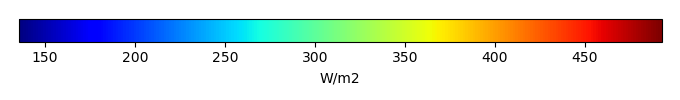MODEL MEANBIAS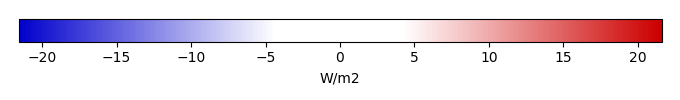BIAS SCORERMSE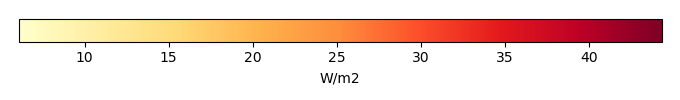RMSE SCOREBENCHMARK INTERANNUAL VARIABILITY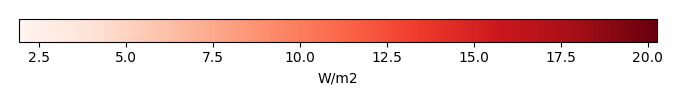MODEL INTERANNUAL VARIABILITYINTERANNUAL VARIABILITY SCOREBENCHMARK MAX MONTHMODEL MAX MONTHDIFFERENCE IN MAX MONTH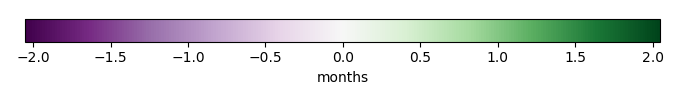SEASONAL CYCLE SCORESPATIAL TAYLOR DIAGRAMMODEL COLORS# Spatially integrated regional mean

MODEL COLORSREGIONAL MEANANNUAL CYCLEMONTHLY ANOMALYANNUAL CYCLE# All Models

BenchmarkCLM5PHSOFFCLM5PHSON# Data Information

creation_date: Thu May 8 23:13:11 PDT 2014

source_file: This product is generated from monthly 1 degree CERES EBAF Radiation observations